# XAT 2022 Question Paper | Quantitative Aptitude and Data Interpretation

###### XAT Previous Year Paper | XAT QADI Questions | Question 23

The best questions to practice for XAT Exam are the actual XAT Question Papers. 2IIM offers you exactly that, in a student friendly format to take value from this. In XAT 2022 we saw some beautiful questions that laid emphasis on Learning ideas from basics and being able to comprehend more than remembering gazillion formulae and shortcuts. Original XAT Question paper is the best place to start off your XAT prep practice. This page provides exactly that. To check out about 1000 CAT Level questions with detailed video solutions for free, go here: CAT Question Bank

Question 23 : Consider the real-valued function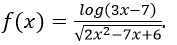.

Find the domain of f(x).

1.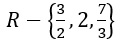2.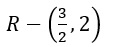3.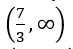4.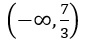5.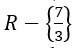## Best CAT Coaching in Chennai

#### CAT Coaching in Chennai - CAT 2022Limited Seats Available - Register Now!

Given that, f(x) = $$frac{$log$3 x-7$}{$sqrt{2 x^{2}-7 x+6}}$ The log values should always be positive. So 3x - 7 > 0 => 3x > 7 Or, x > $$frac{7}{3}$ The value in the root should always be positive and the value in the denominator should not be equal to zero. $2 x^{2}-7 x+6$ > 0$x - 2$(2x - 3) > 0
x < 2 and x > $$frac{3}{2}$ Hence we have x > $$frac{7}{3}$,x < 2 and x > $\frac{3}{2}$ Therefore we can say that x should be always greater than $\frac{7}{3}$ It is represented by$$$frac{7}{3}$,∞$ The question is " Consider the real-valued function. Find the domain of f$x). "

##### Hence, the answer is ''

Choice C is the correct answer.

###### CAT Coaching in ChennaiCAT 2023

Classroom Batches Starting Now! @Gopalapuram

###### Best CAT Coaching in Chennai Introductory offer of 5000/-

Attend a Demo Class

##### Where is 2IIM located?

2IIM Online CAT Coaching
A Fermat Education Initiative,
58/16, Indira Gandhi Street,
Kaveri Rangan Nagar, Saligramam, Chennai 600 093

##### How to reach 2IIM?

Mobile: (91) 99626 48484 / 94459 38484
WhatsApp: WhatsApp Now
Email: info@2iim.com